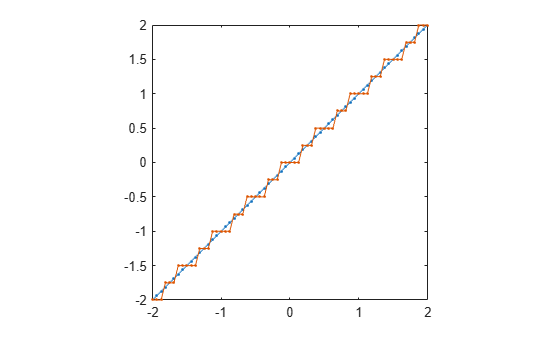# round

Round `fi` object toward nearest integer or round input data using `quantizer` object

## Syntax

``y = round(a)``
``y = round(q,x)``

## Description

example

````y = round(a)` rounds `fi` object `a` to the nearest integer. In the case of a tie, `round` rounds values to the nearest integer with greater absolute value. The rounded value is returned in `fi` object `y`.```

example

````y = round(q,x)` uses the `RoundingMethod` and `FractionLength` settings of `quantizer` object `q` to round the numeric data `x`, but does not check for overflows during the operation. Input `x` must be a built-in numeric variable. Use the `cast` function to work with `fi` objects.```

## Examples

collapse all

The following example demonstrates how the `round` function affects the `numerictype` properties of a signed `fi` object with a word length of 8 and a fraction length of 3.

`a = fi(pi,1,8,3)`
```a = 3.1250 DataTypeMode: Fixed-point: binary point scaling Signedness: Signed WordLength: 8 FractionLength: 3 ```
`y = round(a)`
```y = 3 DataTypeMode: Fixed-point: binary point scaling Signedness: Signed WordLength: 6 FractionLength: 0 ```

The following example demonstrates how the `round` function affects the `numerictype` properties of a signed `fi` object with a word length of 8 and a fraction length of 12.

`a = fi(0.025,1,8,12)`
```a = 0.0249 DataTypeMode: Fixed-point: binary point scaling Signedness: Signed WordLength: 8 FractionLength: 12 ```
`y = round(a)`
```y = 0 DataTypeMode: Fixed-point: binary point scaling Signedness: Signed WordLength: 2 FractionLength: 0 ```

This example shows how to use the rounding method and fraction length specified by `quantizer` object `q` to round the numeric data in `x`.

`q = quantizer('fixed','convergent','wrap',[3 2])`
```q = DataMode = fixed RoundMode = convergent OverflowMode = wrap Format = [3 2] ```
```x = (-2:eps(q)/4:2)'; y = round(q,x); plot(x,[x,y],'.-'); axis square```The functions `convergent`, `nearest`, and `round` differ in the way they treat values whose least significant digit is 5.

• The `convergent` function rounds ties to the nearest even integer.

• The `nearest` function rounds ties to the nearest integer toward positive infinity.

• The `round` function rounds ties to the nearest integer with greater absolute value.

This example illustrates these differences for a given input, a.

```a = fi([-3.5:3.5]'); y = [a convergent(a) nearest(a) round(a)]```
```y = -3.5000 -4.0000 -3.0000 -4.0000 -2.5000 -2.0000 -2.0000 -3.0000 -1.5000 -2.0000 -1.0000 -2.0000 -0.5000 0 0 -1.0000 0.5000 0 1.0000 1.0000 1.5000 2.0000 2.0000 2.0000 2.5000 2.0000 3.0000 3.0000 3.5000 3.9999 3.9999 3.9999 DataTypeMode: Fixed-point: binary point scaling Signedness: Signed WordLength: 16 FractionLength: 13 ```

## Input Arguments

collapse all

Input `fi` array, specified as scalar, vector, matrix, or multidimensional array.

For complex `fi` objects, the imaginary and real parts are rounded independently.

`round` does not support `fi` objects with nontrivial slope and bias scaling. Slope and bias scaling is trivial when the slope is an integer power of 2 and the bias is 0.

Data Types: `fi`
Complex Number Support: Yes

`RoundingMethod` and `FractionLength` settings, specified as a `quantizer` object.

Example: q = quantizer('fixed', 'round', [3 2]);

Input array to quantize using the `quantizer` object `q`, specified as a scalar, vector, matrix, or multidimensional array.

Data Types: `single` | `double` | `int8` | `int16` | `int32` | `int64` | `uint8` | `uint16` | `uint32` | `uint64` | `logical`
Complex Number Support: Yes

## Algorithms

• `y` and `a` have the same `fimath` object and `DataType` property.

• When the `DataType` property of `a` is `single`, `double`, or `boolean`, the `numerictype` of `y` is the same as that of `a`.

• When the fraction length of `a` is zero or negative, `a` is already an integer, and the `numerictype` of `y` is the same as that of `a`.

• When the fraction length of `a` is positive, the fraction length of `y` is `0`, its sign is the same as that of `a`, and its word length is the difference between the word length and the fraction length of `a`, plus one bit. If `a` is signed, then the minimum word length of `y` is `2`. If `a` is unsigned, then the minimum word length of `y` is `1`.

## Extended Capabilities

### HDL Code GenerationGenerate Verilog and VHDL code for FPGA and ASIC designs using HDL Coder™.